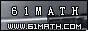Today is . WELCOME: huohai3983/44663  Home | Reg Login | All user | setHomePage | BookmarkHome | Math Test Online | Addition | Subtraction | Multiplication | Division | Time | Worksheet(JPG/PDF) | CAI | Flash Games |Recommendation articleArticle searchHome--maths--Third Grade--Addition of Hours and Minutes

How to add hours and minutes

• If the number of minutes is 60 or more do the following:
• Subtract 60 from the number of minutes.
• Increase the number of hours by one.
• Repeat this process if the number of minutes is still 60 or more.

Example: Add 4 hours 21 minutes plus 2 hours 47 minutes.

• Add the hours together. (4 hours + 2 hours = 6 hours)
• Add the minutes together. (21 minutes + 47 minutes = 68 minutes)
• If the number of minutes is 60 or more do the following:
• Subtract 60 from the number of minutes. (68 minutes - 60 minutes = 8 minutes)
• Increase the number of hours by one. (6 hours + 1 hour = 7 hours)
• Repeat this process if the number of minutes is still 60 or more.
• Answer: 7 hours 8 minutes

#### Find the Total Number of Hours and Minutes

 +   =   hours and   minutes

2006-11-18 22:12:00
Viewed 4174 times CloseGreatmathsites